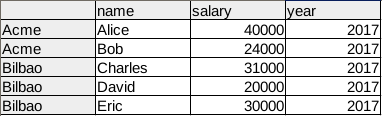# Pandas Filter

Filtering rows of a DataFrame is an almost mandatory task for Data Analysis with Python. Given a Data Frame, we may not be interested in the entire dataset but only in specific rows.

Related course:
Data Analysis in Python with PandasFilter using query
A data frames columns can be queried with a boolean expression. Every frame has the module query() as one of its objects members.

We start by importing pandas, numpy and creating a dataframe:

This will create the data frame containing:After creation of the Data Frame, we call the query method with a boolean expression. This expression is based on the column names that we defined as ‘ABCD’. The query method will return a new filtered data frame.

Total code of data frame creation and filter using boolean expression:

Filter by indexing, chain methods
Instead of queries, we can use in-dices.
We do that by using an array index with boolean expressions:

This will return:

## 2 thoughts on “Pandas Filter”

1.- March 9, 2018

How to run a query involving strings? For Example, print (df.query(‘current_ticket_state’ == ‘Closed’))

1.- March 11, 2018

Here is an example of query involving strings:

`import pandas as pd, numpy as np df = pd.DataFrame({'A': 'foo bar foo bar foo bar foo foo'.split(), 'B': 'one one two three two two one three'.split()}) print(df)print("=============")print( df.query('A == "bar"') )`